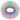## 椭圆内接多边形周长最大值

Mon, 25th November 2019Edit on Githubellipsemaxima周长

# 具体内容

P是已知椭圆上的一点，以P为一顶点且内接于椭圆的n边形中，有且仅有一个周长最长的，记为n(P), 它由下面的2个等价的条件之一决定：
（1） n(P)的全部顶点都具有光反射特性。
（2）n(P)外切于某个与椭圆同焦点的另一个椭圆上。

## 诡异的椭圆定理

hujunhua指出，根据数学星空前面给出的链接（已失效）中结论，应该有$L(4)=4\sqrt{a^2+b^2}$，并且指出，不仅仅是椭圆，对于任意的卵形线，其 周长最大的内接多边形都是反射封闭光路。 他还指出，链接中有一个很有用的命题：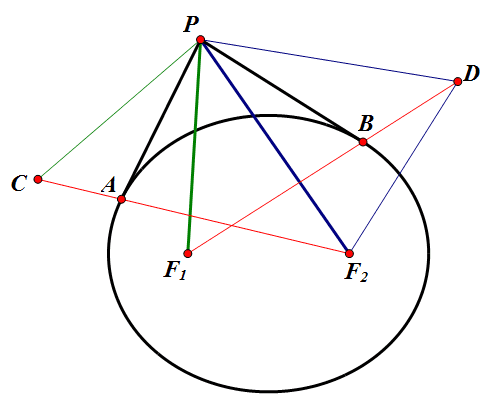hujunhua进一步指出，将$F_1PF_2$想象为一根绷直的绳，两端扎紧在小孔$F_1$$F_2$中，P为一个半径无穷小的张紧轮。当轮P在绳上滚动时，其轨迹形成了椭圆最经典的定义。

mathe建议用$|PA|+|PB|-弧AB$的微分来分析，看看能否证明此微分为0。

zgg__ 建议可以用物理方法分析：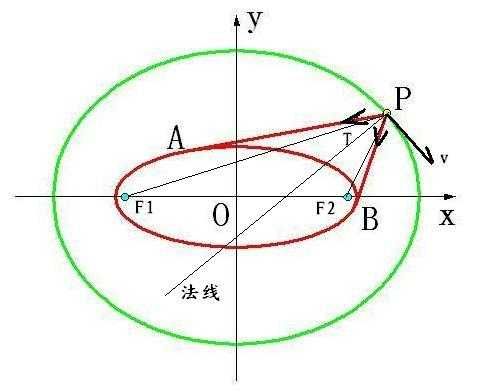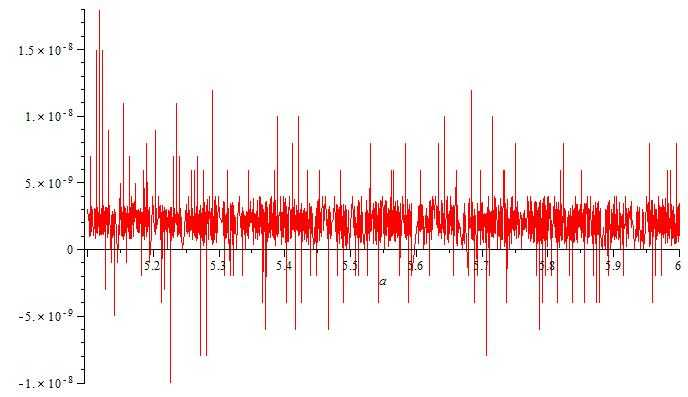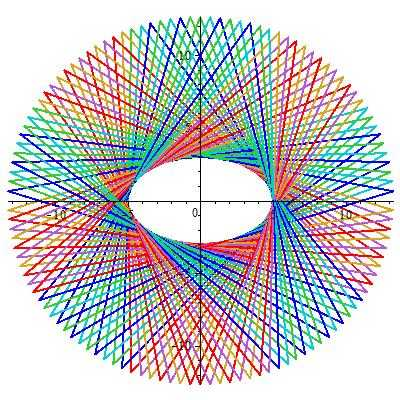zgg__甚至还把问题演绎成非椭圆的一般情况 :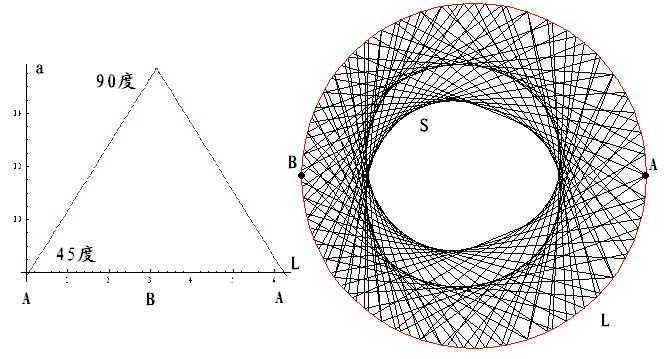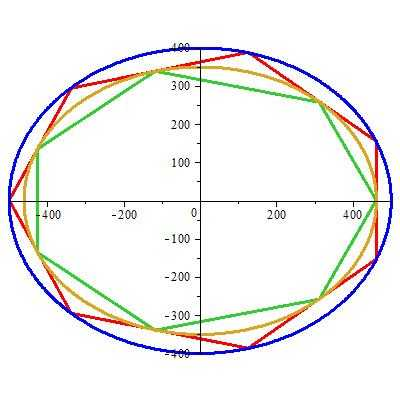kastin也给出了问题运动学证明：

## 引理## 过椭圆外一点Y做两射线与椭圆相切，切点分别为H,K。设距切点最近相应的焦点分别为F1,F2。那么∠F1YH=∠F2YK。

mathe使用在线mathematica中第二类不完全椭圆积分来进行数值计算验证这个问题

wayne使用mathematica进行复杂的计算也验证了这个“诡异的定理”：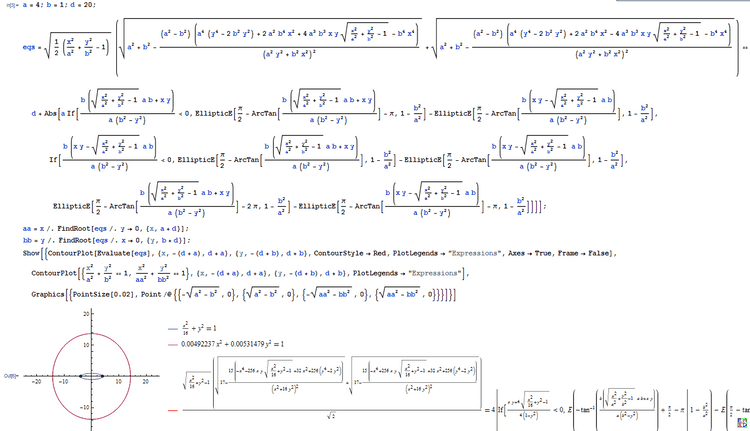mathe建议我们可以改为证明对于一般光滑封闭图形，固定无弹性绳子套作图形移动笔尖做出图形的法向总是俩绳子方向的角平分线，并且给出如下证明：

$\frac{dr}{dt}p\prime(s_2)=\frac{ds_2}{dt}+\frac{dv}{dt}$,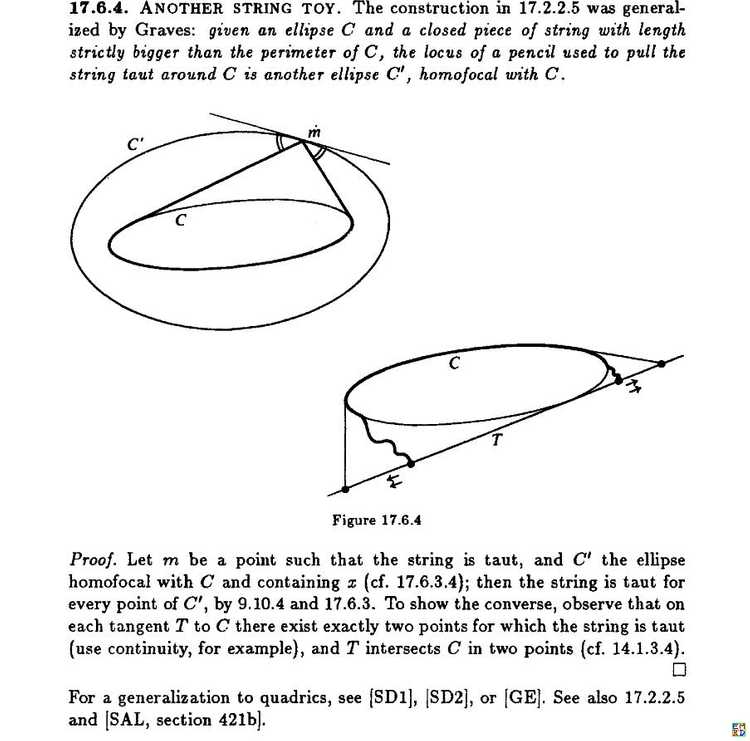## 双心多边形计算

$J=\begin{bmatrix}1&0&0\\0&1&0\\0&0&-r^2\end{bmatrix}$

$K=\begin{bmatrix}\frac1{a^2}&0&-\frac{x_0}{a^2}\\0&\frac1{b^2}&-\frac{y_0}{b^2}\\-\frac{x_0}{a^2}&-\frac{y_0}{b^2}&\frac{x_0^2}{a^2}+\frac{y_0^2}{b^2}-1\end{bmatrix}$

$b^2a^2r^2 x^3 +(−a^2b^2 +a^2y_0^2 −a^2r^2+b^2x_0^2 −b^2r^2 )x^2 +(a^2 −x_0^2+b^2 −y_0^2+r^2)x−1=0$

$x^3-(a^2 −x_0^2+b^2 −y_0^2+r^2)x^2+(a^2b^2 -a^2y_0^2 +a^2r^2 -b^2x_0^2 +b^2r^2)x-a^2b^2r^2=0$

mathe 进一步给出结论：

$\begin{bmatrix}s^2&s&1\end{bmatrix} \begin{bmatrix}a^2b^2&-2ab&1\\-2ab&-2ab+4a+4b-2&-2\\1&-2&1\end{bmatrix} \begin{bmatrix}t^2\\t\\1\end{bmatrix}=0$

$t_1=1,t_2=\frac{4(a-1)(b-1)}{(ab-1)^2}$而且$t_{n+1}=\frac{(t_n-1)^2}{(abt_n-1)^2t_{n-1}}$然后利用$t_n=0$就可以得出n变形情况特征值的约束方程。

## 周长最长内接n边形计算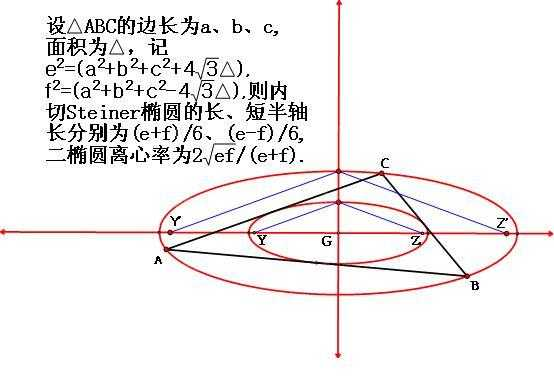$m=a\times\frac{d^2-b^2}{a^2-b^2}$
$n=b\times\frac{a^2-d^2}{a^2-b^2}$
$d^2=\sqrt{a^4+b^4-a^2*b^2}$
$L(3)=\int_0^{2\pi}m\sqrt{1-(m^2-n^2)\frac{\cos(x)^2}{m^2}}dx-3(2\int_0^{arccos(\frac{m}a)}m\sqrt{1-(m^2-n^2)\frac{\cos(x)^2}{m^2}}dx-\frac{2b\sqrt{a^2-m^2}}a$

$L(4)=4\sqrt{a^2+b^2}$
$m=\frac{a^2}{\sqrt{a^2+b^2}}$
$n=\frac{b^2}{\sqrt{a^2+b^2}}$
$L(4)=\int_0^{2\pi}m\sqrt{1-\frac{(m^2-n^2)\cos(x)^2}{m^2}}dx-4(2\int_0^{arccos(\frac ma)}m\sqrt{1-\frac{(m^2-n^2)\cos(x)^2}{m^2}}dx-\frac{2b\sqrt{a^2-m^2}}a$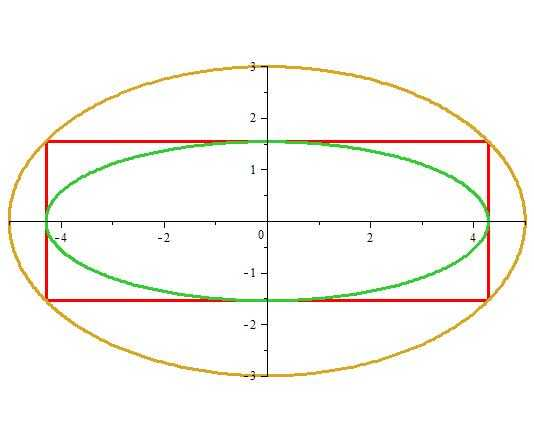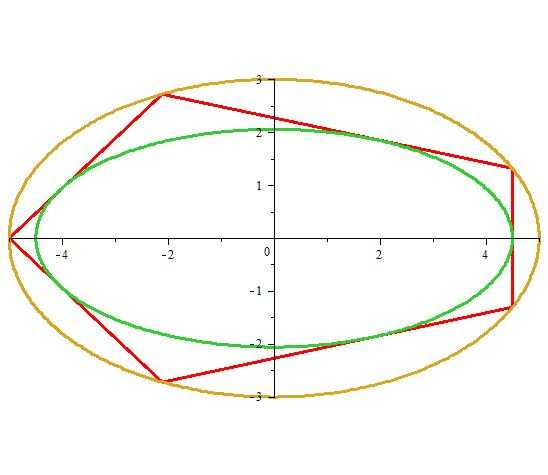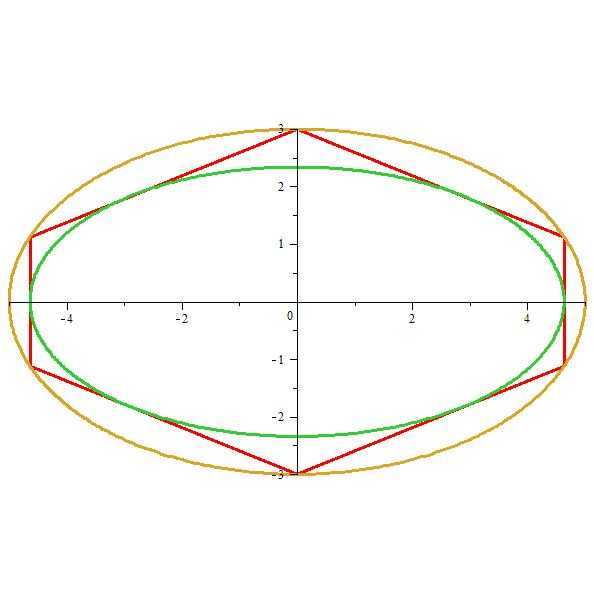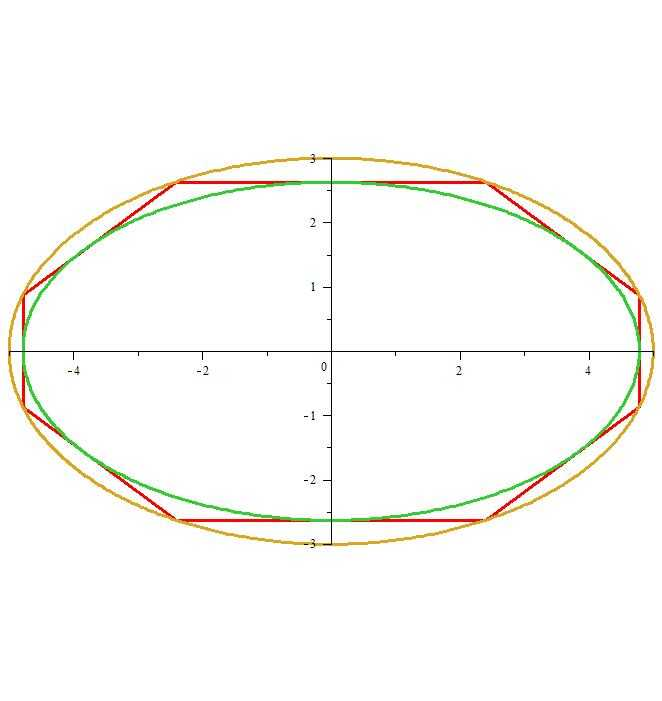Github